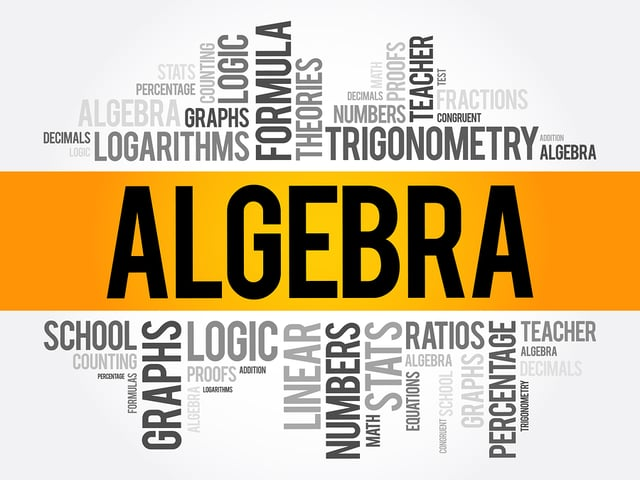# Formulas You’ll Need for the GMAT™ Quantitative Section’s Algebra Questions

Who likes algebra? The perfect student that always sits at the front, or that guy who is crazy about math? What if you can become a lover of algebra? To ace the algebra-related questions in GMAT™ Quantitative Section, you’ll need way more than just “knowing” algebra. You have to develop a close relationship with the procedures and methods for solving algebra problems and, here at Union Test Prep, we want to help you develop that relationship.

The first step in getting close to someone is getting to know everything about them. That’s why, in the following chart, you’ll find the most important algebra formulas you’ll need to get close and personal with algebra. If you use them to solve the FREE problems we also have for you here at Union Test Prep, we’re sure you won’t dread Algebra anymore, but, instead, you may even long for her!

You’ll find formulas relating to the other types of questions on this section of the test here:

Arithmetic and Geometry

Statistics and Problem Solving

## Algebra Formulas

Category Formula Symbols Comment
General
Algebra
$$x+a=b \Rightarrow x=b-a$$
$$x-a=b \Rightarrow x=b+a$$
$$x \cdot a=b \Rightarrow x=b \div a$$
$$x \div a=b \Rightarrow x=b \cdot a$$
$$x^a=b \Rightarrow x = \sqrt[a]{b}$$
$$\sqrt[a]{x}= b \Rightarrow x= b^a$$
$$a^x=b \Rightarrow x=\frac{log\ b}{log\ a}$$
a, b = constants
x = variable

General
Algebra
$$x^a \cdot x^b = x^{a+b}$$ a, b, x = any real number
General
Algebra
$$\frac{x^a}{x^b}=x^{a-b}$$ a, b, x = any real number
General
Algebra
$$(x^a)^b = x^{a \cdot b}$$ a, b, x = any real number
General
Algebra
$$(x \cdot y)^a = x^a \cdot y^a$$ a, b, x = any real number
General
Algebra
$$x^1 = x$$ x = any real number
General
Algebra
$$x^0 = 1$$ x = any real number
General
Algebra
$$x^{-a} = \frac {1}{x^a}$$ a, x = any real number
General
Algebra
$$x^{\frac {a}{b}} = \sqrt[b]{x^a} = (\sqrt[b]{x})^a$$ a, b, x = any real number
General
Algebra
$$\frac{x}{\sqrt{y}} \cdot \frac {\sqrt{y}}{\sqrt{y}} = \frac{x \sqrt{y}}{y}$$ x, y = any real number
Linear
Equations
$$A \cdot x + B \cdot y = C$$ A, B, C = any real number
y = dependent variable
x = independent variable
Standard form
Linear
Equations
$$y = m \cdot x + b$$ y = dependent variable
m = slope
x = independent variable
b = y axis intercept
Slope-intercept form
Try to convert any linear
equation to this form.
Linear
Equations
$$m = \frac{(y_2 - y_1)}{(x_2 - x_1)}$$ m = slope
$$y_n$$ = independent variable (point n)
$$x_n$$ = dependent variable (point n)
This is a rearrangement of the
point-slope form.
Linear
Equations
$$y-y_1 = m(x-x_1)$$ $$(x_1,y_1)$$ = point on the line
m = slope
y = independent variable
x = dependent variable
Point-slope form
Equations
$$x= \frac{-b \pm \sqrt{b^2-4 \cdot a \cdot c}}{2 \cdot a}$$ a, b, c = constants
c = y axis intercept
x = variable
equation in the form
$$a^2+bx+c=0$$
$$(a \pm b)^2 = a^2 \pm 2 \cdot a \cdot b + b^2$$ a, b = constants or variables Square of a sum or difference
$$a^2 - b^2 = (a-b) \cdot (a+b)$$ a, b = constants or variables Difference of two squares
$$a^3 - b^3 = (a-b) \cdot (a^2+ab+b^2)$$ a, b = constants or variables Difference of two cubes
$$a^3 + b^3 = (a+b) \cdot (a^2-ab+b^2)$$ a, b = constants or variables Sum of two cubes